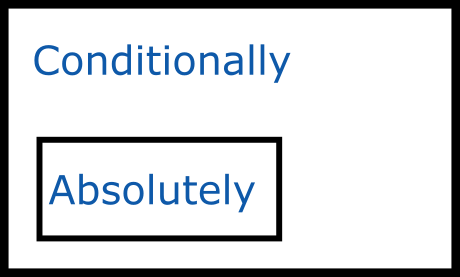Show Question
Math and science::Analysis::Tao::07. Series

# Absolute convergence, and the absolute convergence test

### Absolute convergence, definition

Let $$\sum_{n=m}^{\infty}a_n$$ be a formal series of real numbers. The series is said to be absolutely convergent if the series $$\sum_{n=m}^{\infty}|a_n|$$ is convergent.

In order to distinguish convergence from absolute convergence, we sometimes refer to the former as conditional convergence.

### Absolute convergence test

Let $$\sum_{n=m}^{\infty}a_n$$ be a formal series of real numbers. If the series is absolutely convergent, then it is also conditionally convergent. Furthermore, in this case we have the triangle inequality

$\left| \sum_{n=m}^{\infty}a_n \right| \le \sum_{n=m}^{\infty}|a_n|$

Note that the converse of this proposition is not true; there exists series that are conditionally convergent but are not absolutely convergent.p167-168